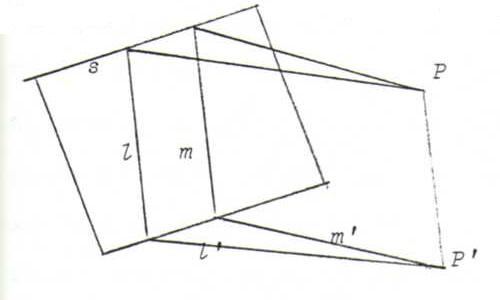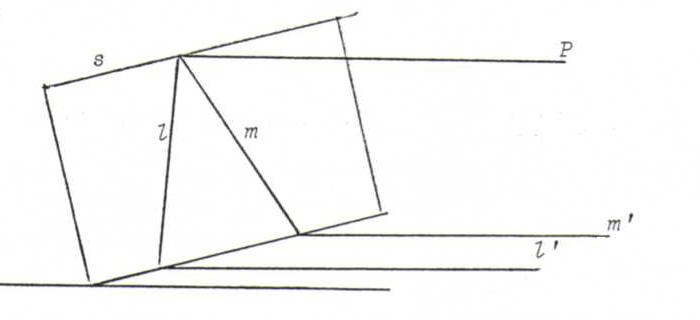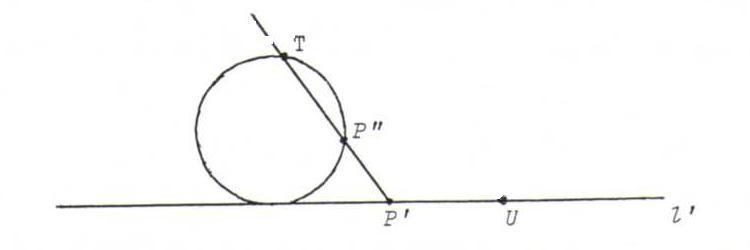### COURSE OF PROJECTIVE GOMETRY

§ 1: answers

O1iii) (see the picture above) l' and m' intersect in the point of projection of P on α (direction of projection l).iv) (see the picture above) l' and m' are parallel to the line that connects P and the point of intersection of l and m on s.

O2 All concepts mentioned above except distance and angular measure.
Example: if l and m in β are parallel, then so are their images l' and m' under parallel projection onto α.

O3 PP" where P" is the other point of intersection of TP' and c (T at the top of c).The point U where l' intersects u has no original under the central projection, so the other point of intersection of TU and c has no original.
Furthermore, T itself has no original, either.
After reading the next section you will understand that the central projection maps the point at infinity of l onto U, and that the mapping of this problem maps S onto T.
You can see that the mapping is continuous if you formulate continuity in terms of neighbourhoods (of the proper points and of the point at infinity on a line).

O4 The tangents that touch c in A and B correspond to the asymptotes of the hyperbola.
The lines through A or B in β correspond to the lines in α that have an asymptotical direction.
Because these lines intersect c in at most one point that doesn't lie on s, their image lines intersect the hyperbola in at most one (proper) point.